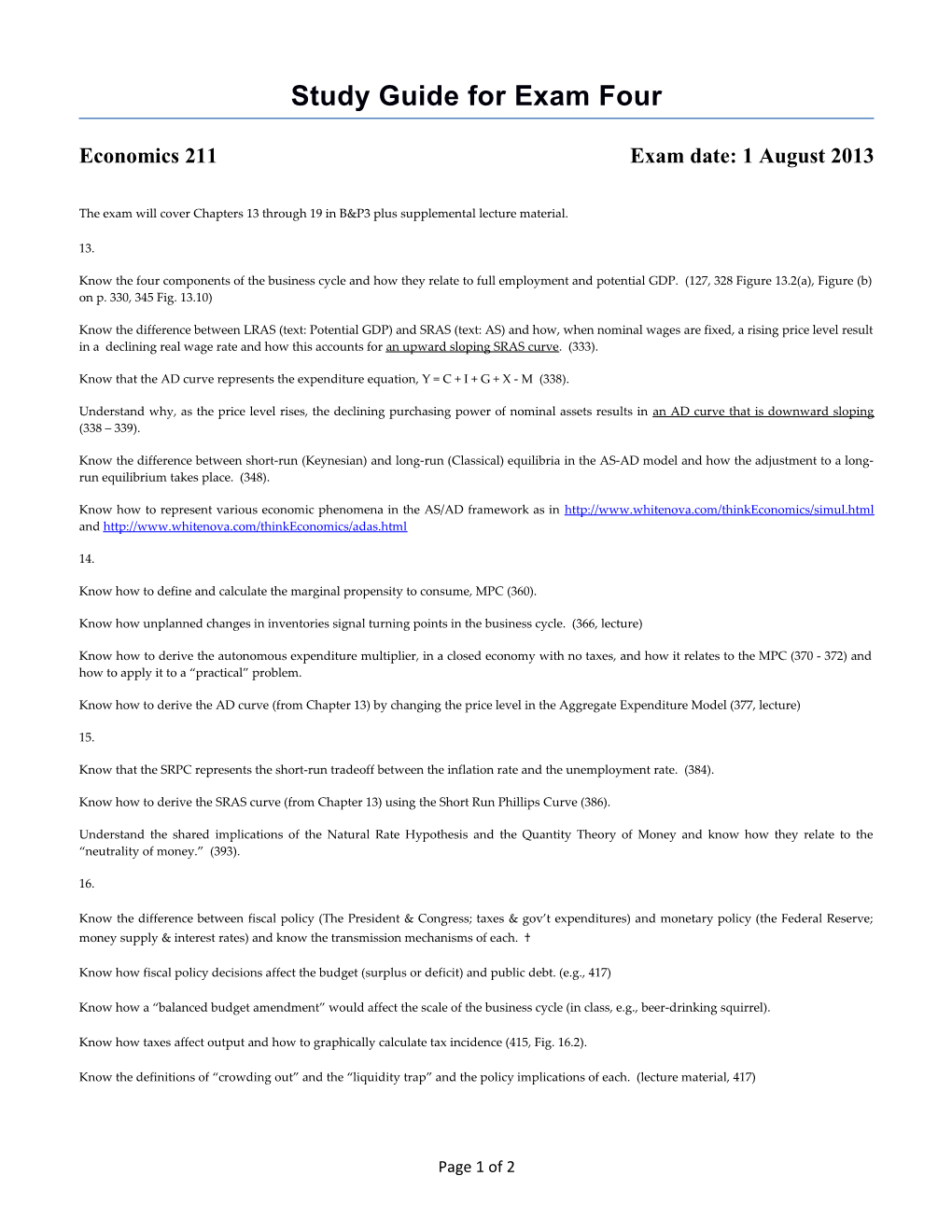# Study Guide for Exam FourStudy Guide for Exam Four

Economics 211 / Exam date: 1 August 2013

The exam will cover Chapters 13 through 19 in B&P3 plus supplemental lecture material.

13.

Know the four components of the business cycle and how they relate to full employment and potential GDP. (127, 328 Figure 13.2(a), Figure (b) on p. 330, 345 Fig. 13.10)

Know the difference between LRAS (text: Potential GDP) and SRAS (text: AS) and how, when nominal wages are fixed, a rising price level result in a declining real wage rate and how this accounts foran upward sloping SRAS curve. (333).

Know that the AD curve represents the expenditure equation, Y = C + I + G + X - M (338).

Understand why, as the price level rises, the declining purchasing power of nominal assets results in an AD curve that is downward sloping (338 – 339).

Know the difference between short-run (Keynesian) and long-run (Classical) equilibria in the AS-AD model and how the adjustment to a long-run equilibrium takes place. (348).

Know how to represent various economic phenomena in the AS/AD framework as in and

14.

Know how to define and calculate the marginal propensity to consume, MPC (360).

Know how unplanned changes in inventories signal turning points in the business cycle. (366, lecture)

Know how to derive the autonomous expenditure multiplier,in a closed economy with no taxes, and how it relates to the MPC (370 - 372) and how to apply it to a “practical” problem.

Know how to derive the AD curve (from Chapter 13) by changing the price level in the Aggregate Expenditure Model (377, lecture)

15.

Know that the SRPC represents the short-run tradeoff between the inflation rate and the unemployment rate. (384).

Know how to derive the SRAS curve (from Chapter 13) using the Short Run Phillips Curve (386).

Understand the shared implications of the Natural Rate Hypothesis and the Quantity Theory of Money and know how they relate to the “neutrality of money.” (393).

16.

Know the difference between fiscal policy (The President Congress; taxes gov’t expenditures) and monetary policy (the Federal Reserve; money supply interest rates) and know the transmission mechanisms of each. †

Know how fiscal policy decisions affect the budget (surplus or deficit) and public debt. (e.g., 417)

Know how a “balanced budget amendment” would affect the scale of the business cycle (in class, e.g., beer-drinking squirrel).

Know how taxes affect output and how to graphically calculate tax incidence (415, Fig. 16.2).

Know the definitions of “crowding out” and the “liquidity trap” and the policy implications of each. (lecture material, 417)

Know how monetary and fiscal policy decisions can impact AD and AS (in class and and how to represent various economic conditions using the AS-AD model (in class and

† Know especially the transmission mechanism of monetary policy as per (the Fed eases) and (the Fed tightens).

17.

Know how to derive a money multiplier similar to the one on p. 290 of the text but which accounts for the growing importance of excess reserves. (in class)

Know how AS-AD equilibrates under both Keynesian and monetarist scenarios. (§18.2 in ch18lecture.ppt, Rules v. Discretion)

Know how monetary policy decisions affect the price level---i.e., the Quantity Theory of Money (311-313)

Know how the policy objectives and open market operations differ between the Federal Reserve System and the European Central Bank. (in class)

18.

Understand how gains from trade can be achieved. (75-77, 469-472)

Have a general understanding of the key organizations which promote international trade and how they function, especially the WTO.

Understand the effects of tariffs and quotas. (476-481)

Know the key arguments and counter arguments for trade restrictions. (483-487)

19.

Know the balance of payments accounts and, in particular, the symmetry of the capital account with current account. (494-495)

Know the factors which can shift the demand or supply of foreign exchange. (502-508)

Know the definitions and meaning of purchasing power parity (PPP),

where S is the exchange of currency A to currency B, is the cost of basket X in currency A, and is the cost of basket X in currency B. (510, lecture material)

Know the definitions and meaning of naked and covered interest rate parity (IRP),

and

respectively, where is the k-period interest rate in country A, is the k-period interest rate in country B, is the spot exchange rate at time t, is the k-period futures contract for S at time t, andis the expected value of at time t; and know how IRP relates to the currency carry trade. (512, lecture material)

Page 1 of 1